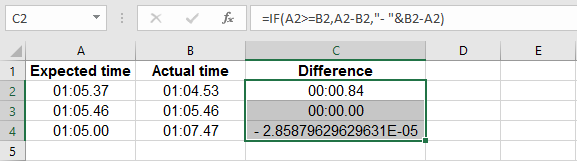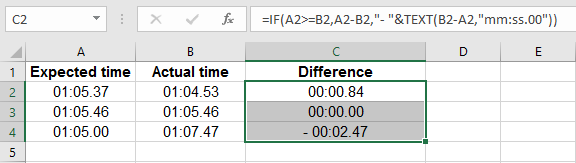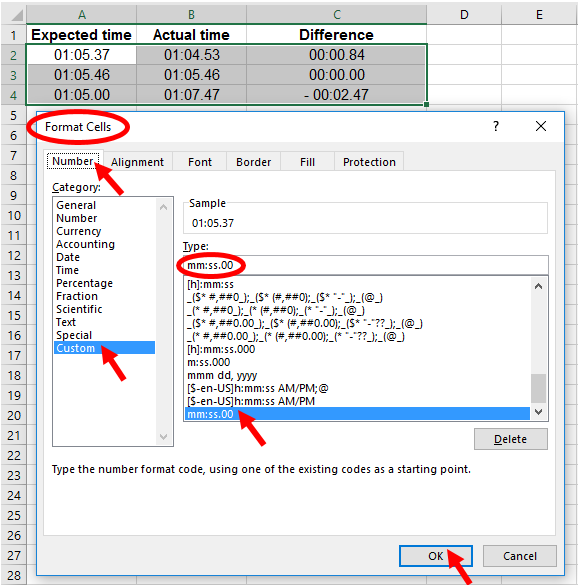Calculating Negative Time Differences in Hundredths of a Second - EBOOK VBA EXCELEVBA.info ( Ebook VBA Excel ) Welcome EVBA.info - Nothing Is Unable ... About Excel Tricks, Learning VBA Programming, Dedicated Software, Free Courses, Living Skills ...

## Tuesday, December 10, 2019

Calculating Negative Time Differences in Hundredths of a Second
When you want to calculate differences in time when the measurements are in hundredths of a second, special consideration must be given for cases when the result is negative. The next pictures show examples of calculations when the Actual time is less than expected; exactly as expected, and more than expected.
If every calculation was to have a positive result, you could use the formula
`=IF(A2 > =B2,A2-B2,"- "&B2-A2)`
A problem happens when, as shown in cell C4 using that formula, the result is a negative number displayed in an unintuitive format.The next picture shows the first formula revised with the `TEXT` function:
`=IF(A2 > =B2,A2-B2,"- "&TEXT(B2-A2,"mm:ss.00"))`The cells are custom-formatted `mm:ss.00`📤You download App EVBA.info installed directly on the latest phone here : https://www.evba.info/p/app-evbainfo-setting-for-your-phone.html?m=1